# Linear Algebra - Point

Zero-dimensional: Span of the empty set: The origin

A point (x, y) can be represented in the plane by a {'x','y'}-vector$\begin{bmatrix} x \\ y \end{bmatrix}$ .

A point can also be represented by {'x','y','z'}-vector$\begin{bmatrix} x \\ y \\ z\end{bmatrix}$ . This representation is called homogeneous coordinates

Discover MoreData Visualization - Vector Graphic

A vector graphic is a composed of geometric object and is next to raster (bitmap Image) the second way of representing a image It represents object not by their pixel as in raster (bitmap Image) but by...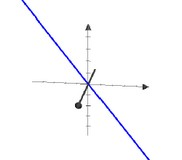Geometry - Object

in Geometry An object can be: a pre-defined shape or an arbitrary one defined with the help of a path. Notions such as: The length of a vector The angle between two vectors. are introduced...Geometry - Origin

All geometrics objects definition goes through the origin. In order to go through an another point, a translation (addition) must be performed.Geometry - Shape (with transformation and notion)

in Geometry A shape is any list of line drawing commands, which strung together, describe a continuous line or closed shape that can be filled or stroked. Move, Line, Quadratic and Cubic...Linear Algebra - Linear combination

A linear combination of the vectors is the following expression: where: The scalars are the coefficients Every vector in is a linear combination: Every linear combination is a vector in...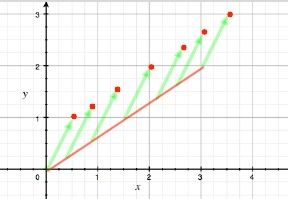Linear Algebra - (Line|Line Segment)

in linear algebra. Line and line segment definition in Linear Algebra that goes: A line has a dimension of one because only one coordinate is needed to specify a point on it Line_segmentline...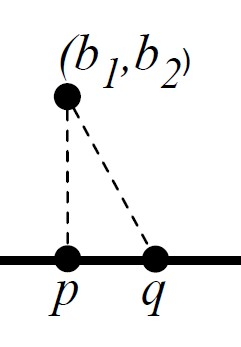Linear Algebra - Closest Point on a Line

How to find the closest point on a line from a point ? How to find the vector on the line that best approximates the given vector b (the closest point on the line) Letb be a vector Leta be a...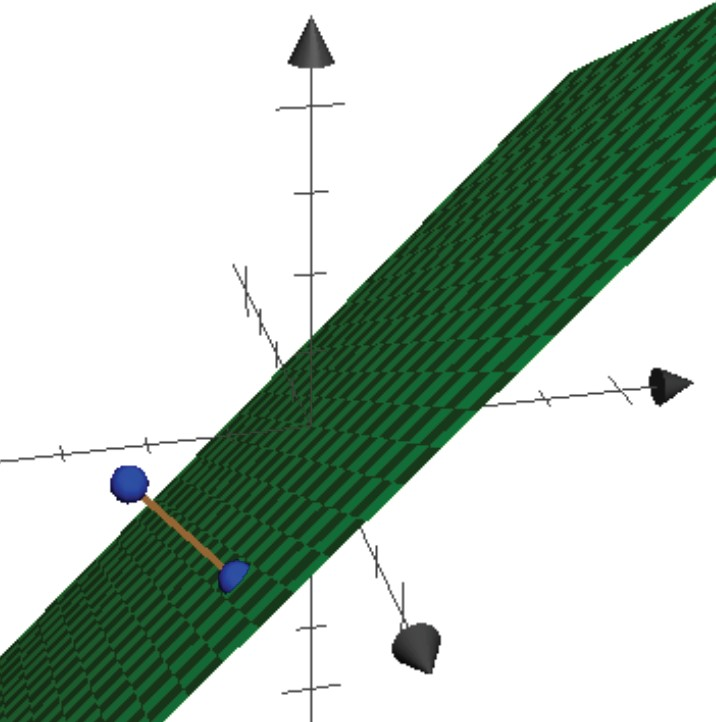Linear Algebra - Closest point in a plane

Given a point b and a plane, find the point in the plane closest to b. By , we can assume the plane includes the origin. The plane is a vector space V. Let {v1, v2} be a basis for V. Goal: Given...Linear Algebra - Coordinate system

coordinate system in terms of vector. Idea of coordinate system for a vector space V: (and generalized beyond two dimensions), Coordinate system for a vector space V is specified by generators of...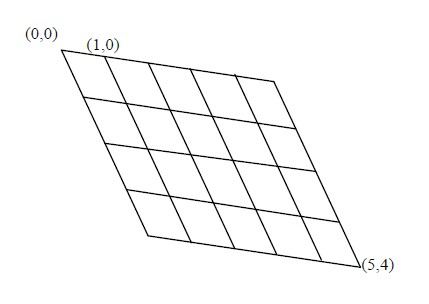Linear Algebra - Image representation

A generalized image consists of a grid of generalized pixels, where each generalized pixel is a quadrilateral (not necessarily a rectangle). Think of an image as a grid of rectangles, each assigned a...